Fluid Properties

# Fluid Properties - Notes | Study Mechanical Engineering SSC JE (Technical) - Mechanical Engineering

 1 Crore+ students have signed up on EduRev. Have you?

FLUID PROPERTIES

Definition of Fluids:- A fluid is a substance which flow continuously under the action of shear force (however small the force may be), as long as shear force is there the fluid moves or deforms.
E.g: - Liquids, gases, vapour etc.
For a static fluid shear force = 0

• Density or mass density (S):- Mass per unit volume of a fluid is called its density
s = (m/v)
• Density of water is maximum at 4ºC.
• Density of water at 4ºC = 1000 kg/m3.

• Ideal Fluid (Perfect Fluid)
• Non-viscous, friction less & incompressible.
• Does not offer shear resistance against flow.
• Bulk modulus is infinite
• Used in mathematical analysis and flow problems.
• Ideal fluid has no surface tension

• Real Fluid
• Possess the properties such as viscosity, surface tension and compressibility.
• Offers resistance against flow.

• Specific gravity (G or S)
• G or S = Density of substance / Density of water at 40C
• Specific gravity for water is 1.0 at 4ºC and  that of mercury is 13.6
• Specific gravity varies with temperature therefore it should be determined at specified temperature (4ºC)

• Specific weight (unit weight) or weight density (g')
g'=rg
r = mass density
g = due to gravity, acceleration

Newton's Law of Viscosity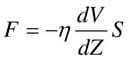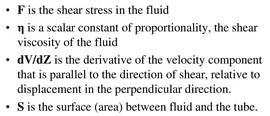Non-Newtonian Fluids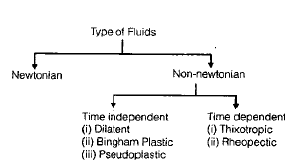• These do not follow Newton's law of viscosity. The relation between shear sterss and velocity gradient is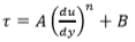Where A and B are constants depending upon type of fluid and condition of flow.(i) For Dilatant Fluids: n > 1 & B = 0
Ex. Butter, Quick sand
(ii) For Bingham Plastic Fluids: n = 1 & B ¹ 0 Ex. Sewage sludge, Drilling mud These fluids always have certain minimum shear stress before they yield.
(iii) For Pseudoplastic Fluids: n < 1 & B = 0 Ex. Paper pulp, Rubber solution, Lipsticks, Paints, Blood, Polymetric solution etc.
(iv) For Thixotropic Fluids : n < 1 & B ¹ 0 Viscosity increase with time.
Ex. Printers ink and Enamels.
(v) For Rheopectic Fluids: n > 1 & B ¹ 0 Viscosity decreases with time
Ex. Gypsum solution in water & Bentonite solution.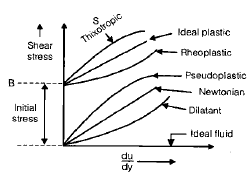• Viscosity
• Due to viscosity a fluid offers resistance to flow. It is due to cohesion and intermolecular attraction.
(i) Dynamic Viscosity ( m) Its SI unit is Pascal second or N-s/m2 Its CGS unit is Poise = Dyne-sec/cm2 1 poise = 0.1 N–s/m2
(ii) Kinematic Viscosity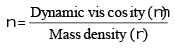Its SI unit is m2/s.
Its CGS unit is cm2/s or stoke
1 Stoke = 10–4 m2/s

• Viscosity of liquids decrease with temperature whereas viscosity of gases increase with temperature.
• fluids with increasing order of viscosity are air, gasoline, water, crude oil, castor oil.
• Viscosity of water at 1ºc is 1 centipoise.
• Viscosity of fluids is due to cohesion and molecular momentum transfer.

• Surface Tension (S)
• Surface tensions is due to cohesion only.
• Surface tension decrease with increase in temperature and it alos act when fluid is at rest
• It is a surface phenomenon
• At critical point liquid and vapour are at same state thus suface tension is zero swater = 0.0736 N/m at 20ºC
Smercury = 4.51 N/m
• (i) Pressure inside a liquid drop, in excess of atmospheric pressure

p= 4s/d,                                                                                                                                             where s is surface tension d is dia. of drop

• (ii)Pressure inside a soap bubble, in excess of atmospheric pressure
p= 8s/d
• (iii) Pressure inside jct

p = 2s/d

• Capillary Action
• Capillary action is due to adhesion and cohesion, both.
• Height of water in capillary tube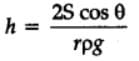where,
g = Specific weight,
h = rise in capillary

ρ = density of fluid
S = surface tension of water & glass
θ = angle of contact between the liquid and the material
θ — 0º for water and glass
θ — 128º for mercury and glass

For capillary action dia of tube should be less than 3cm.

• When a liquid surface supports another liquid of density " rb ", then rise in capillary is given as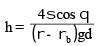Compressibility

• It refers to change in volume/density due to change in pressure.
• The compressibility reciprocal of bulk modulus of elasticity (K).

Compressibility  = 1/k

• In compressible fluids the velocity of sound is given by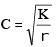C = velocity of sound in fluid
K = Bulk modulus of fluid

The document Fluid Properties - Notes | Study Mechanical Engineering SSC JE (Technical) - Mechanical Engineering is a part of the Mechanical Engineering Course Mechanical Engineering SSC JE (Technical).
All you need of Mechanical Engineering at this link: Mechanical Engineering

## Mechanical Engineering SSC JE (Technical)

6 videos|97 docs|55 tests

## Mechanical Engineering SSC JE (Technical)

6 videos|97 docs|55 tests

Track your progress, build streaks, highlight & save important lessons and more!

,

,

,

,

,

,

,

,

,

,

,

,

,

,

,

,

,

,

,

,

,

;